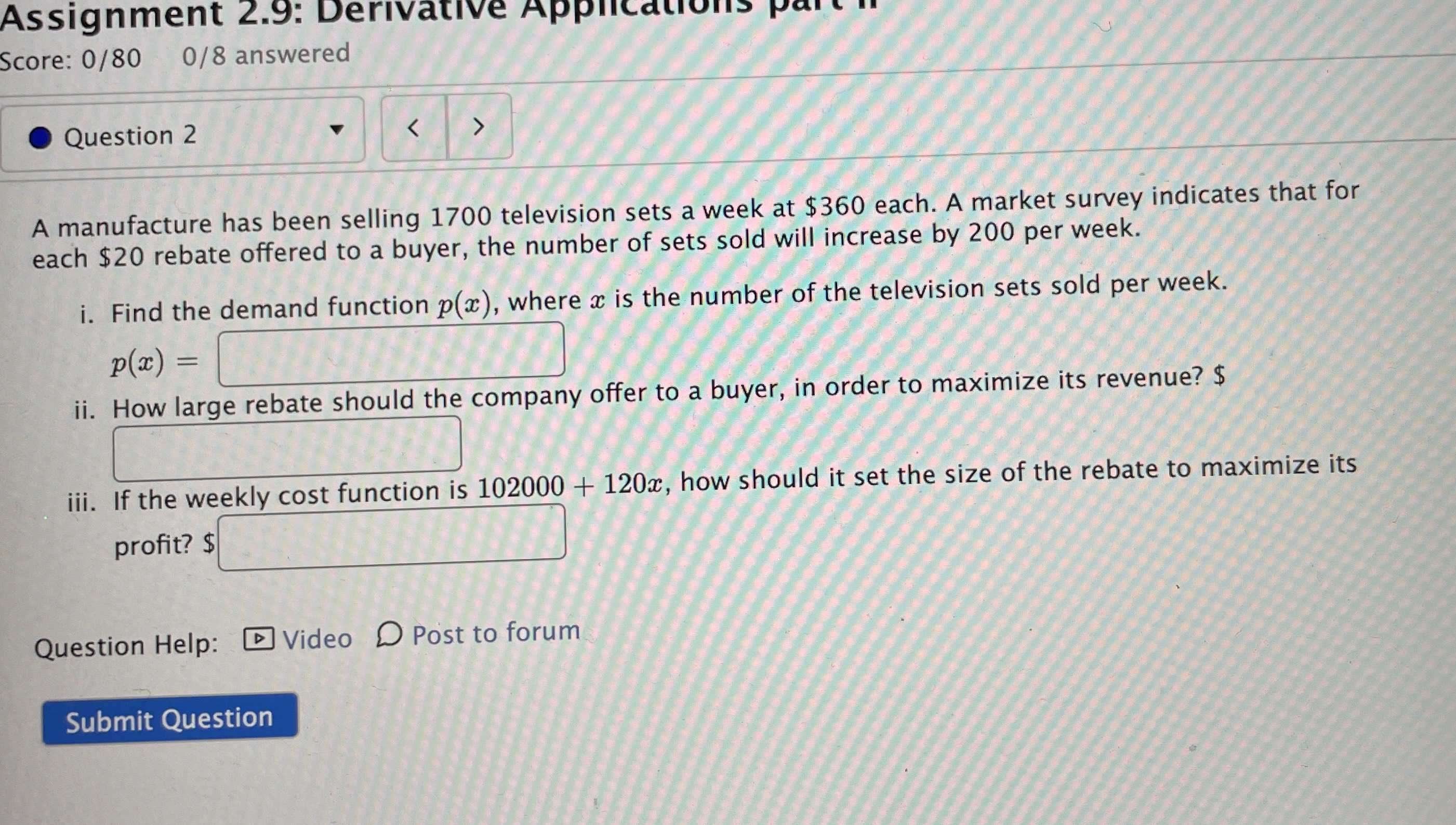### ¿Todavía tienes preguntas de matemáticas?

Pregunte a nuestros tutores expertos
Algebra
PreguntaA manufacture has been selling $$1700$$ television sets a week at $$\ 360$$ each. A market survey indicates that for each $$\ 20$$ rebate offered to a buyer, the number of sets sold will increase by $$200$$ per week.

i. Find the demand function $$p ( x )$$ , where $$x$$ is the number of the television sets sold per week.

$$p ( x ) =$$

ii. How large rebate should the company offer to a buyer, in order to maximize its revenue?

iii. If the weekly cost function is $$102000 + 120 x$$ , how should it set the size of the rebate to maximize its profit?

-$$\frac{x}{10}$$+530